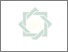# Determinate factors of mathematics problem solving ability toward spatial, verbal and mathematical logic intelligence aspects

Kusaeri, Kusaeri and Sholeh, B. (2016) Determinate factors of mathematics problem solving ability toward spatial, verbal and mathematical logic intelligence aspects. In: Ideas for 21st Century Education: Proceedings of the Asian Education Symposium (AES 2016), 22-23 November 2016, Bandung - Indonesia.Preview
Text
Determinate factors of mathematics problem solving ability toward spatial.pdf

## Abstract

This research aimed to know and to analyze the direct and indirect effects of three multi- ple intelligences (spatial, verbal, mathematical-logic intelligences) towards students’ mathematical problem solving ability. The subjects were 280 of 9th grade students of SMP Negeri 37 Surabaya. The data were collected by mathematical logic intelligence, problem solving ability, and psychological tests. The psy- chological test was used to measure psychological constructs of spatial and verbal intelligences. The data analysis used inferential statistics, path analysis. The results showed that: (1) the mathematical logic intel- ligence was the first determinant factor in the students’ ability in mathematical problem solving (42.7%), and the second was the verbal intelligence (29.2%); (2) the verbal and mathematical logic intelligences had a direct effect to the ability of mathematical problem solving, simultaneously. The spatial intelligence affected indirectly the ability of mathematical problem solving. Individually or simultaneously, it appears that verbal and mathematical logic intelligences had a direct effect to the ability of mathematical problem solving. It implies that mathematics teachers of SMP/MTS should give more opportunities and stimulus of mathematical logic problems and verbal abilities to their students.

## Statistic

Item Type: Conference or Workshop Item (Paper)
Creators:
CreatorsEmailNIM
Kusaeri, Kusaerikusaeri@uinsby.ac.idUNSPECIFIED
Sholeh, B.--UNSPECIFIED
Subjects: Matematika
Keywords: Spatial; verbal; mathematical logic intelligence
Divisions: Karya Ilmiah > Prosiding
Depositing User: Editor : Abdun Nashir------ Information------library.uinsby.ac.id
Date Deposited: 11 May 2018 02:41View Item